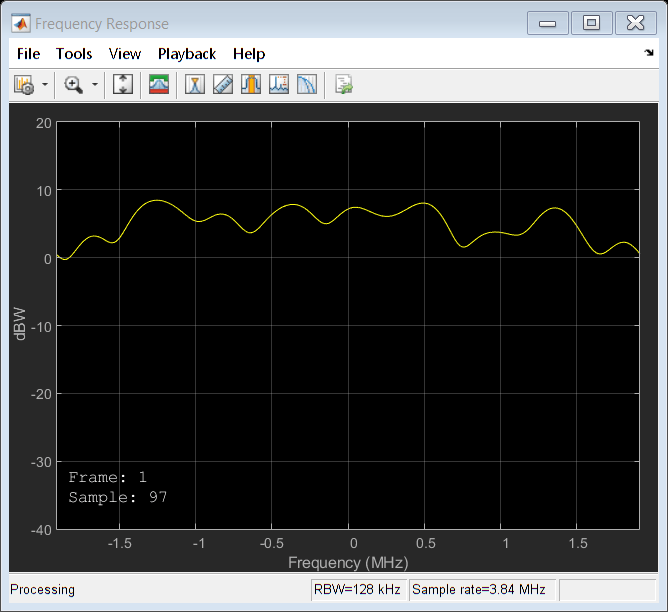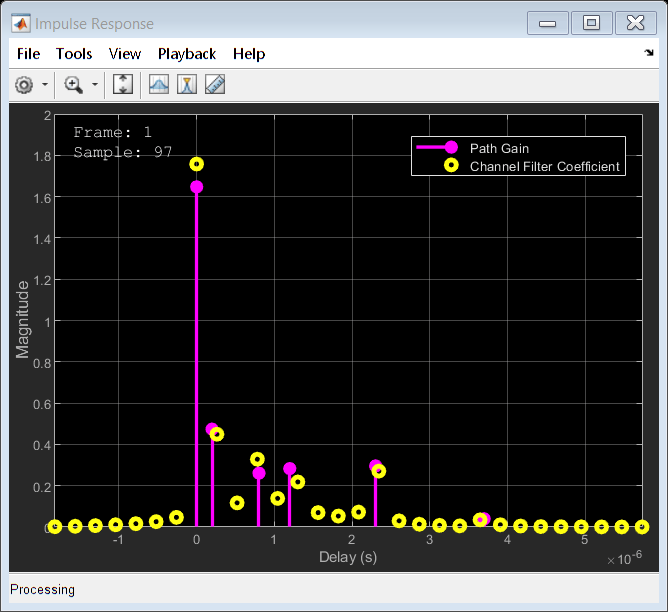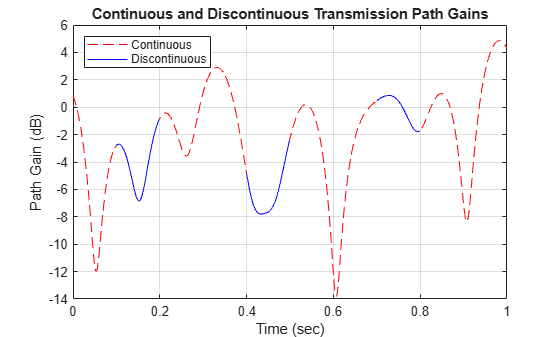# comm.RayleighChannel

Filter input signal through multipath Rayleigh fading channel

## Description

The `comm.RayleighChannel` System object™ filters an input signal through a multipath Rayleigh fading channel. For more information on fading model processing, see Methodology for Simulating Multipath Fading Channels.

To filter an input signal using a multipath Rayleigh fading channel:

1. Create the `comm.RayleighChannel` object and set its properties.

2. Call the object with arguments, as if it were a function.

## Creation

### Syntax

``rayleighchan = comm.RayleighChannel``
``rayleighchan = comm.RayleighChannel(Name,Value)``

### Description

````rayleighchan = comm.RayleighChannel` creates a frequency-selective or frequency-flat multipath Rayleigh fading channel System object. This object filters a real or complex input signal through the multipath channel to obtain the channel-impaired signal.```

example

````rayleighchan = comm.RayleighChannel(Name,Value)` sets properties using one or more name-value pair arguments. Enclose each property name in quotes. For example, `comm.RayleighChannel('SampleRate',2)` sets the input signal sample rate to 2.```

## Properties

expand all

Unless otherwise indicated, properties are nontunable, which means you cannot change their values after calling the object. Objects lock when you call them, and the `release` function unlocks them.

If a property is tunable, you can change its value at any time.

Input signal sample rate in Hz, specified as a positive scalar.

Data Types: `double`

Discrete path delay in seconds, specified as a numeric scalar or numeric row vector.

• When `PathDelays` is a scalar, the channel is frequency flat.

• When `PathDelays` is a vector, the channel is frequency selective.

Data Types: `double`

Average gains of the discrete paths in decibels, specified as a numeric scalar or numeric row vector. `AveragePathGains` must be of the same size as the `PathDelays` property.

Data Types: `double`

Normalize average path gains to 0 dB, specified as a logical `1` (`true`) or `0` (`false`).

• When `NormalizePathGains` is `true`, the fading processes are normalized so that the total power of the path gains, averaged over time, is 0 dB.

• When `NormalizePathGains` is `false`, the total power of the path gains is not normalized.

Data Types: `logical`

Maximum Doppler shift for all channel paths, specified as a nonnegative scalar. Units are in hertz.

The maximum Doppler shift limit applies to each channel path. When you set this property to `0`, the channel remains static for the entire input. You can use the `reset` object function to generate a new channel realization. The `MaximumDopplerShift` property value must be smaller than `SampleRate`/10/fc for each path. fc represents the cutoff frequency factor of the path. For most Doppler spectrum types, the value of fc is 1. For Gaussian and biGaussian Doppler spectrum types, fc is dependent on the Doppler spectrum structure fields. For more details about how fc is defined, see Cutoff Frequency Factor.

Data Types: `double`

Doppler spectrum shape for all channel paths, specified as a Doppler spectrum structure or a 1-by-NP cell array of Doppler spectrum structures. These Doppler spectrum structures must be outputs of the form returned from the `doppler` function. NP is the number of discrete delay paths, that is, the length of the `PathDelays` property.

• When `DopplerSpectrum` is defined by a single Doppler spectrum structure, all paths have the same specified Doppler spectrum.

• When `DopplerSpectrum` is defined by a cell array of Doppler spectrum structures, each path has the Doppler spectrum specified by the corresponding structure in the cell array.

Options for the spectrum type are specified by the `specType` input to the `doppler` function. If you set the `FadingTechnique` property to ```'Sum of sinusoids'```, you must set `DopplerSpectrum` to `doppler('Jakes')`.

#### Dependencies

To enable this property, set the `MaximumDopplerShift` property to a positive value. The `MaximumDopplerShift` property defines the maximum Doppler shift value permitted when specifying the Doppler spectrum.

Data Types: `struct` | `cell`

Channel model fading technique, specified as ```'Filtered Gaussian noise'``` or `'Sum of sinusoids'`.

Data Types: `char` | `string`

Number of sinusoids used to model the fading process, specified as a positive integer.

#### Dependencies

To enable this property, set the `FadingTechnique` property to `'Sum of sinusoids'`.

Data Types: `double`

Source to control the start time of the fading process, specified as `'Property'` or `'Input port'`.

• When `InitialTimeSource` is set to `'Property'`, use the `InitialTime` property to set the initial time offset.

• When `InitialTimeSource` is set to ```'Input port'```, specify the start time of the fading process by using the `itime` input to the System object. The input value can change in consecutive calls to the System object.

#### Dependencies

To enable this property, set the `FadingTechnique` property to `'Sum of sinusoids'`.

Data Types: `string` | `char`

Initial time offset, in seconds, for the fading model, specified as a nonnegative scalar. `InitialTime` must be greater than end time of the last frame. `InitialTime` is rounded up to the nearest sample position when it is not a multiple of 1/`SampleRate`.

#### Dependencies

To enable this property, set the `FadingTechnique` property to `'Sum of sinusoids'` and the `InitialTimeSource` property to `'Property'`.

Data Types: `double`

Source of random number stream, specified as `'Global stream'` or `'mt19937ar with seed'`.

• `'Global stream'` — The current global random number stream is used for normally distributed random number generation. In this case, the `reset` object function resets the channel filters only.

• `'mt19937ar with seed'` — The mt19937ar algorithm is used for normally distributed random number generation. In this case, the `reset` object function resets the channel filters and reinitializes the random number stream to the value of the `Seed` property.

Data Types: `string` | `char`

Initial seed of mt19937ar random number stream generator algorithm, specified as a nonnegative integer. When the `reset` object function is called, the mt19937ar random number stream is reinitialized to the `Seed` property value.

#### Dependencies

To enable this property, set the `RandomStream` property to `'mt19937ar with seed'`.

Data Types: `double`

Option to output path gains, specified as `0` (`false`) or `1` (`true`). Set this property to `1` (`true`) to output the channel path gains of the underlying fading process.

Data Types: `logical`

Channel visualization, specified as `'Off'`, ```'Impulse response'```, `'Frequency response'`, ```'Impulse and frequency responses'```, or `'Doppler spectrum'`. For more information, see Channel Visualization.

Data Types: `string` | `char`

Percentage of samples to display, specified as `'25%'`, `'10%'`, `'50%'`, or `'100%'`. Displaying fewer samples improves (decreases) the display update rate at the expense of decreasing the visualized precision.

#### Dependencies

To enable this property, set the `Visualization` property to `'Impulse response'`, ```'Frequency response'```, or ```'Impulse and frequency responses'```.

Data Types: `string` | `char`

Path for Doppler display, specified as a positive integer. Use this property to select the discrete path used in constructing a Doppler spectrum plot. The specified path must be an element of {1, 2, ..., NP}. In this set, NP is the number of discrete delay paths, that is, the length of the `PathDelays` property value.

#### Dependencies

To enable this property, set the `Visualization` property to `'Doppler spectrum'`.

Data Types: `double`

## Usage

### Syntax

``y = rayleighchan(x)``
``[y,pathgains] = rayleighchan(x)``
``___ = rayleighchan(x,itime)``

### Description

````y = rayleighchan(x)` filters input signal `x` through a multipath Rayleigh fading channel and returns the output signal in `y`.```
````[y,pathgains] = rayleighchan(x)` returns the channel path gains of the underlying multipath Rayleigh fading process in `pathgains`. To enable this syntax set the `PathGainsOutputPort` property set to `1` (`true`).```
````___ = rayleighchan(x,itime)` passes data through the multipath Rayleigh fading channel beginning at the initial time specified by `itime`. To enable this syntax, set the `FadingTechnique` property to ```'Sum of sinusoids'``` and the `InitialTimeSource` property to `'Input port'`.```

### Input Arguments

expand all

Input signal, specified as an NS-by-1 numeric vector, where NS is the number of samples.

Data Types: `single` | `double`
Complex Number Support: Yes

Initial time in seconds, specified as a nonnegative scalar. The `itime` input must be greater than the end time of the last frame. When `itime` is not a multiple of 1/`SampleRate`, it is rounded up to the nearest sample position.

Data Types: `single` | `double`

### Output Arguments

expand all

Output signal, returned as an NS-by-1 numeric vector of complex values with the same data precision as the input signal `x`. NS is the number of samples.

Path gains, returned as an NS-by-NP array. NS is the number of samples. NP is the number of discrete delay paths, that is, the length of the `PathDelays` property value. `pathgains` contains complex values with the same precision as the input signal `x`.

## Object Functions

To use an object function, specify the System object as the first input argument. For example, to release system resources of a System object named `obj`, use this syntax:

`release(obj)`

expand all

 `info` Characteristic information about fading channel object
 `step` Run System object algorithm `release` Release resources and allow changes to System object property values and input characteristics `reset` Reset internal states of System object

## Examples

collapse all

This example shows how to produce the same multipath Rayleigh fading channel response by using two different methods for random number generation. The multipath Rayleigh fading channel System object™ includes two methods for random number generation. You can use the current global stream or the mt19937ar algorithm with a specified seed. By interacting with the global stream, the System object can produce the same outputs from these two methods.

Create a PSK modulator System object to modulate randomly generated data.

```pskModulator = comm.PSKModulator; channelInput = pskModulator(randi([0 pskModulator.ModulationOrder-1],1024,1));```

Create a multipath Rayleigh fading channel System object, specifying the random number generation method as the my19937ar algorithm and the random number seed as 22.

```rayleighchan = comm.RayleighChannel(... 'SampleRate',10e3, ... 'PathDelays',[0 1.5e-4], ... 'AveragePathGains',[2 3], ... 'NormalizePathGains',true, ... 'MaximumDopplerShift',30, ... 'DopplerSpectrum',{doppler('Gaussian',0.6),doppler('Flat')}, ... 'RandomStream','mt19937ar with seed', ... 'Seed',22, ... 'PathGainsOutputPort',true);```

Filter the modulated data by using the multipath Rayleigh fading channel System object.

`[chanOut1,pathGains1] = rayleighchan(channelInput);`

Set the System object to use the global stream for random number generation.

```release(rayleighchan); rayleighchan.RandomStream = 'Global stream';```

Set the global stream to have the same seed that was specified when creating the multipath Rayleigh fading channel System object.

`rng(22)`

Filter the modulated data by using the multipath Rayleigh fading channel System object again.

`[chanOut2,pathGains2] = rayleighchan(channelInput);`

Verify that the channel and path gain outputs are the same for each of the two methods.

`isequal(chanOut1,chanOut2)`
```ans = logical 1 ```
`isequal(pathGains1,pathGains2)`
```ans = logical 1 ```

This example shows how to create a frequency-selective multipath Rayleigh fading channel System object and display its impulse and frequency responses.

Set the sample rate to 3.84 MHz. Specify path delays and gains by using the ITU pedestrian B channel configuration. Set the maximum Doppler shift to 50 Hz.

```fs = 3.84e6; % Hz pathDelays = [0 200 800 1200 2300 3700]*1e-9; % sec avgPathGains = [0 -0.9 -4.9 -8 -7.8 -23.9]; % dB fD = 50; % Hz```

Create a multipath Rayleigh fading channel System object by using the previously defined properties and to visualize the impulse response and frequency response plots.

```rayleighchan = comm.RayleighChannel('SampleRate',fs, ... 'PathDelays',pathDelays, ... 'AveragePathGains',avgPathGains, ... 'MaximumDopplerShift',fD, ... 'Visualization','Impulse and frequency responses');```

Generate random binary data and pass it through the multipath Rayleigh fading channel. The impulse response plot enables you to identify the individual paths and their corresponding filter coefficients. The frequency response plot shows the frequency-selective nature of the ITU pedestrian B channel.

```x = randi([0 1],1000,1); y = rayleighchan(x);```The example shows how the channel state is maintained in cases in which data is discontinuously transmitted. Create a multipath Rayleigh fading channel System object and pass data through it using the sum-of-sinusoids technique.

Set the channel properties.

```fs = 1000; % Sample rate (Hz) pathDelays = [0 2.5e-3]; % Path delays (s) pathPower = [0 -6]; % Path power (dB) fD = 5; % Maximum Doppler shift (Hz) ns = 1000; % Number of samples nsdel = 100; % Number of samples for delayed paths```

Define the overall simulation time and three time segments for which data is to be transmitted. In this case, the channel is simulated for 1s with a 1000 Hz sampling rate. One 1000-sample continuous data sequence is transmitted at time 0. Three 100-sample data packets are transmitted at times 0.1 s, 0.4 s, and 0.7 s, respectively.

```to0 = 0.0; to1 = 0.1; to2 = 0.4; to3 = 0.7; t0 = (to0:ns-1)/fs; % Transmission 0 t1 = to1+(0:nsdel-1)/fs; % Transmission 1 t2 = to2+(0:nsdel-1)/fs; % Transmission 2 t3 = to3+(0:nsdel-1)/fs; % Transmission 3```

Generate random binary data corresponding to the previously defined time intervals.

```d0 = randi([0 1],ns,1); d1 = randi([0 1],nsdel,1); d2 = randi([0 1],nsdel,1); d3 = randi([0 1],nsdel,1);```

Create a frequency-flat multipath Rayleigh fading System object, specifying the sum-of-sinusoids fading technique. So that results can be repeated, specify a seed value. Use the default `InitialTime` property setting so that the fading channel will be simulated from time 0. Enable the path gains output.

```rayleighchan1 = comm.RayleighChannel('SampleRate',fs, ... 'MaximumDopplerShift',5, ... 'RandomStream','mt19937ar with seed', ... 'Seed',17, ... 'FadingTechnique','Sum of sinusoids', ... 'PathGainsOutputPort',true);```

Create a clone of the multipath Rayleigh fading channel System object. Set the source for initial time so that the fading channel offset time can be specified as an input argument when using the System object.

```rayleighchan2 = clone(rayleighchan1); rayleighchan2.InitialTimeSource = 'Input port';```

Pass random binary data through the first multipath Rayleigh fading channel System object, `rayleighchan1`. Data is transmitted over all 1000 time samples. For this example, only the complex path gain is needed.

`[~,pg0] = rayleighchan1(d0);`

Pass random data through the second multipath Rayleigh fading channel System object, `rayleighchan2`, where the initial time offsets are provided as input arguments.

```[~,pg1] = rayleighchan2(d1,to1); [~,pg2] = rayleighchan2(d2,to2); [~,pg3] = rayleighchan2(d3,to3);```

Compare the number of samples processed by the two channels by using the `info` object function. The `rayleighchan1` object processed 1000 samples, while the `rayleighchan2` object only processed 300 samples.

```G = info(rayleighchan1); H = info(rayleighchan2); [G.NumSamplesProcessed H.NumSamplesProcessed]```
```ans = 1×2 1000 300 ```

Convert the path gains into decibels.

```pathGain0 = 20*log10(abs(pg0)); pathGain1 = 20*log10(abs(pg1)); pathGain2 = 20*log10(abs(pg2)); pathGain3 = 20*log10(abs(pg3));```

Plot the path gains for the continuous and discontinuous cases. The gains for the three segments match the gain for the continuous case. The alignment of the two highlights that the sum-of-sinusoids technique is suited to the simulation of packetized data, as the channel characteristics are maintained even when data is not transmitted.

```plot(t0,pathGain0,'r--') hold on plot(t1,pathGain1,'b') plot(t2,pathGain2,'b') plot(t3,pathGain3,'b') grid xlabel('Time (sec)') ylabel('Path Gain (dB)') legend('Continuous','Discontinuous','location','nw') title('Continous and Discontinuous Transmission Path Gains')```The example show how to use the `ChannelFilterCoefficients` property returned by the `info` object function of the `comm.RayleighChannel` System object to reproduce the multipath Rayleigh fading channel output.

Create a multipath Rayleigh fading channel System object, defining two paths and specifying data to pass through the channel.

```rayleighchan = comm.RayleighChannel('SampleRate',1000,'PathDelays',[0 1.5e-3], ... 'AveragePathGains',[0 -3],'PathGainsOutputPort',true)```
```rayleighchan = comm.RayleighChannel with properties: SampleRate: 1000 PathDelays: [0 0.0015] AveragePathGains: [0 -3] NormalizePathGains: true MaximumDopplerShift: 1.0000e-03 DopplerSpectrum: [1x1 struct] Show all properties ```
`data = randi([0 1],600,1);`

Pass data through the channel. Assign the `ChannelFilterCoefficients` property value to the variable `coeff`.

```[chanout1,pg] = rayleighchan(data); chaninfo = info(rayleighchan)```
```chaninfo = struct with fields: ChannelFilterDelay: 6 ChannelFilterCoefficients: [2x16 double] NumSamplesProcessed: 600 ```
`coeff = chaninfo.ChannelFilterCoefficients;`

Calculate the fractional delayed input signal at the path delay locations stored in `coeff`.

```Np = length(rayleighchan.PathDelays); fracdelaydata = zeros(size(data,1),Np); for ii = 1:Np fracdelaydata(:,ii) = filter(coeff(ii,:),1,data); end```

Apply the path gains and sum the results for all paths.

`chanout2 = sum(pg .* fracdelaydata,2);`

Compare the output of the multipath Rayleigh fading channel System object to the output reproduced using the path gains and the `ChannelFilterCoefficients` property of the multipath Rayleigh fading channel System object.

`isequal(chanout1,chanout2)`
```ans = logical 1 ```

expand all

 Oestges, Claude, and Bruno Clerckx. MIMO Wireless Communications: From Real-World Propagation to Space-Time Code Design. 1st ed. Boston, MA: Elsevier, 2007.

 Correia, Luis M., and European Cooperation in the Field of Scientific and Technical Research (Organization), eds. Mobile Broadband Multimedia Networks: Techniques, Models and Tools for 4G. 1st ed. Amsterdam ; Boston: Elsevier/Academic Press, 2006.

 Kermoal, J.P., L. Schumacher, K.I. Pedersen, P.E. Mogensen, and F. Frederiksen. “A Stochastic MIMO Radio Channel Model with Experimental Validation.” IEEE Journal on Selected Areas in Communications 20, no. 6 (August 2002): 1211–26. https://doi.org/10.1109/JSAC.2002.801223.

 Jeruchim, Michel C., Philip Balaban, and K. Sam Shanmugan. Simulation of Communication Systems. Second edition. Boston, MA: Springer US, 2000.

 Patzold, M., Cheng-Xiang Wang, and B. Hogstad. “Two New Sum-of-Sinusoids-Based Methods for the Efficient Generation of Multiple Uncorrelated Rayleigh Fading Waveforms.” IEEE Transactions on Wireless Communications 8, no. 6 (June 2009): 3122–31. https://doi.org/10.1109/TWC.2009.080769.

﻿